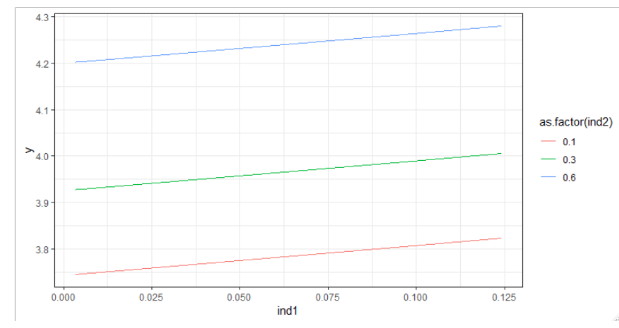# How to perform sensitivity analysis?

693    Asked by DanPeters in Data Science , Asked on Jun 1, 2020

Suppose after log transformation we have following equation

Now we will be doing sensitivity analysis and create a graph where x axis is first independent variable (ind1), y axis is y where we will plot different curvatures of 2nd independent value

This is our original data

df <- data.frame(y, ind1, Ind2)

structure(list(y = c(17.5460, 15.09292, 404.6266, 509.8268,

22.29624, 27.62826), ind1 = c(0.003739, 0.003473,

0.09648630.12409780.005281, 0.005089), Ind2 = c(0.20708,

0.216378, 0.628712, 0.505681, 0.318622, 0.2451923 )),

row.names = c(NA, 6L), class = "data.frame")

# Vector of values over which to perform the analysis.

vec.pars <- c(0.1, 0.3, 0.6)

# The function for calculating Y.

calcY <- function(x, ind1) {

3.651 + 0.648 * ind1 + 0.915 * x

}

# Vectorized version. This enables us to apply the above function

# to a vector of values at the same time.

calcY <- Vectorize(FUN = calcY, vectorize.args = "x", SIMPLIFY = FALSE)

# Construct the output

vec.y <- calcY(x = vec.pars, ind1 = df\$ind1)

xy <- data.frame(y = do.call(c, vec.y))

xy\$ind1 <- rep(df\$ind1, times = length(vec.y))

xy\$ind2 <- rep(vec.pars, each = nrow(df))

ggplot(xy, aes(x = ind1, y = y, color = as.factor(ind2))) +

theme_bw() +

geom_line()

Output: